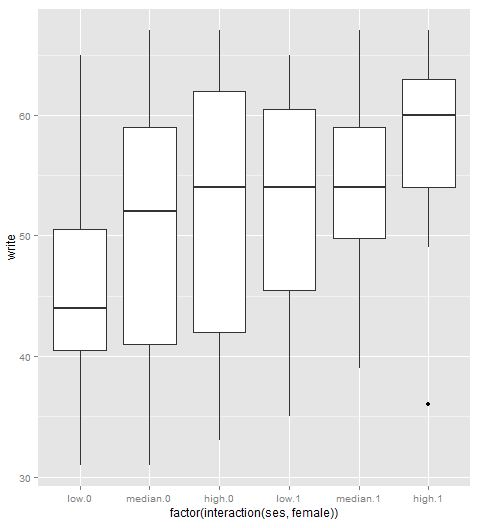## Sunday, December 9, 2012

### R: Replicate plot with ggplot2 (part 3) boxplot

This is to replicate the boxplot from UCLA ATS:　Examples of box plots

First read in the data:

The first is to draw the boxplot with no featuresThe second is filling the box area with blue color.The third is the box plot splited by a categorical variable ses:Next is to rename the levels of the categorical variable:The fifth one is to set notch = TRUEThe sixth is the plot on the interaction levels of more than one categorical variable:A little more, if we want to add outliers on the plot, it should be like: